Students can download Maths Chapter 4 Geometry Ex 4.4 Questions and Answers, Notes, Samacheer Kalvi 9th Maths Guide Pdf helps you to revise the complete Tamilnadu State Board New Syllabus, helps students complete homework assignments and to score high marks in board exams.

## Tamilnadu Samacheer Kalvi 9th Maths Solutions Chapter 4 Geometry Ex 4.4

Question 1.
Find the value of x in the given figure.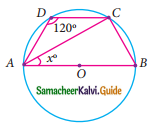Solution:
∠B = 180° – 120°
(Sum of the opposite angles of a quadrilateral are supplementary)
∠B = 60°
∠BCA = 90° (Angle in a semicircle)
∠BAC + ∠B + ∠BCA = 180°
x + 60° + 90° = 180°
x + 150° = 180°
x = 180° – 150°
= 30°
The value of x = 30°Question 2.
In the given figure, AC is the diameter of the circle with centre O.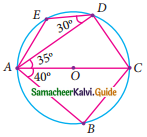If ∠ADE = 30°; ∠DAC = 35° and ∠CAB = 40°.
Find (i) ∠ACD
(ii) ∠ACB
(iii) ∠DAE
Solution:
(i) ∠ADC = 90° (Angle in a semi-circle)
∠ADC + ∠ACD + ∠DAC = 180° (Sum of the angles of a triangle is 180°)
90° + ∠ACD + 35° = 180°
∠ACD = 180° – 125°
∠ACD = 55°

(ii) ∠ABC = 90° (Angle in a semi-circle)
∠ACB + ∠CBA + ∠BAC = 180° (Sum of the angles of a triangle is 180°)
∠ACB + 90 °+ 40° = 180°
∠ACB = 180° – 130°
= 50°

(iii) In the cyclic quadrilateral ACDE,
∠AED = 180° – 55°
= 125°
In ΔAED,
∠DAE + ∠AED + ∠EDA = 180° (Sum of the angles of a triangle is 180°)
∠DAE + 125° + 30° = 180°
∠DAE = 180°- 155°
= 25°
∴ ∠DAE = 25°Question 3.
Find all the angles of the given cyclic quadrilateral ABCD in the figure.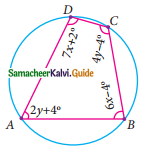Solution:
∠B + ∠D = 180° (Sum of the opposite angles of a cyclic quadrilateral is 180°)
6x – 4° + 7x + 2° = 180°
13x – 2° = 180°
13x = 182°
x = 182°
x = $$\frac{182°}{13}$$
x = 14°
∠B = 6x – 4°
= 6(14) – 4°
= 84 – 4
= 80°
∠D = 7x + 2°
7(14) + 2°
98 + 2
= 100°
180°
2y + 4° + 4y – 4° = (Sum of the opposite angles of a cyclic quadrilateral is 180°)
6y = 180°
y = $$\frac{180°}{6}$$
= 30°
∠A = 2y + 4°
= 2(30) + 4°
= 64°
∠C = 4y – 4°
= 4(30) – 4°
= 120° – 4°
= 116°
∴ ∠A = 64°, ∠B = 80°, ∠C = 116°, ∠D = 100°.Question 4.
In the given figure, ABCD is a cyclic quadrilateral where diagonals intersect at P such that ∠DBC = 40° and ∠BAC = 60° find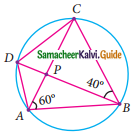(ii) ∠BCD
Solution:
(i) ∠CAD = ∠DBC = 40° [Angles at the circumference to the same segment]
(ii) ∠BAC – ∠BDC = 60° [Angles at the circumference to the same segment]
∠BCD + ∠BDC + ∠CBD = 180° (Sum of the three angles of A is 180°)
∠BCD + 60° + 40° = 180°
∠BCD = 180° – 100°
= 80°Question 5.
In the given figure, AB and CD are the parallel chords of a circle with centre O. Such that AB = 8 cm and CD = 6 cm. If OM ⊥ AB and OL ⊥ CD distance between LM is 7cm. Find the radius of the circle?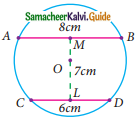Solution:
Let OM be x.
∴ OL = 7 – x
In the right ΔAOM,
OA² = AM² + OM²
= 4² + x²
OA² = 16 + x²
r² = 16 + x² ……… (1) [r is the radius]
In the right ΔOCL,
OC² = OL² + CL²
r² = (7 – x)² + 3²
= 49 + x² – 14x + 9
= 58 + x² – 14x …….. (2)
From (1) and (2) we get,
16 + x² = 58 + x² – 14x
14x = 58 – 16
14x = 42
x = $$\frac{42}{14}$$
x = 3 cm
r² = 16 + x²
= 16 + 9
= 25
∴ r = $$\sqrt{25}$$
= 5
∴ radius of the circle = 5 cm.Question 6.
The arch of a bridge has dimensions as shown, where the arch measure 2 m at its highest point and its width is 6 cm. What is the radius of the circle that contains the arch?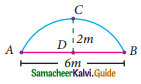Solution:
AD = $$\frac{6}{2}$$
= 3 m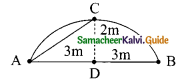= 32 + 22
= 9 + 4
= 13
AC = $$\sqrt{13}$$
= 3.6mQuestion 7.
In figure ∠ABC = 120°, where A, B and C are points on the circle with centre O. Find ∠OAC?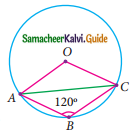Solution:
Reflex ∠AOC = 2∠ABC
= 20 × 120°
= 240°
∴ ∠AOC = 360° – 240°
= 120°
∠OCA + ∠OAC = 180° – 120°
= 60°
∴ ∠OAC = $$\frac{60}{2}$$
= 30° (Since OA = OC)Question 8.
A school wants to conduct tree plantation programme. For this a teacher allotted a circle of radius 6m ground to nineth standard students for planting sapplings. Four students plant trees at the points A, B, C and D as shown in figure. Here AB = 8m, CD = 10m and AB ⊥ CD. If another student places a flower pot at the point P, the intersection of AB and CD, then find the distance from the centre to P.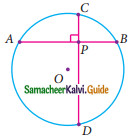Solution:
OA = OD = 6 m
AB = 8 m (chord)
CD = 10 m (chord)
In Δ AOM, OM = $$\sqrt{6² – 4²}$$ (∴ OM bisects the chord and ⊥ to the chord)
= $$\sqrt{36 – 16}$$
= $$\sqrt{20}$$m
In Δ CON, ON = $$\sqrt{6² – 5²}$$
= $$\sqrt{36 – 25}$$
= $$\sqrt{11}$$m (ON bisects the chord and ⊥ to the chord)
ONPM is a rectangle with all the angles 90° and with length $$\sqrt{20}$$ m, breadth $$\sqrt{11}$$l m.
We need to find OP which is the diagonal of the rectangle ONPM.
∴ OP = $$\sqrt{ON² + NP²}$$ = $$\sqrt{(\sqrt{11})² + (\sqrt{20})²}$$
(∴ OM = NP, opposite sides of the rectangle)
= $$\sqrt{11 + 20}$$
= $$\sqrt{31}$$
= 5.56 mQuestion 9.
In the given figure, ∠POQ = 100° and ∠PQR° = 30°, then find ∠RPO.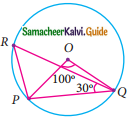Solution:
∠PRQ = $$\frac{1}{2}$$ ∠POQ (Angles at the circumference)
= $$\frac{1}{2}$$ × 100°
= 50°
∠OPQ + ∠OQP = 180° – 100° (Total angles of A is 180°)
= 80°
∴ ∠OPQ = $$\frac{80}{2}$$
= 40° (Since OP = OQ, radius of the circle)
∠RPQ = 180°- (30 + 50)°
= 100°
∴ ∠RPO = ∠RPQ – ∠OPQ
= 100° – 40°
= 60°